机器学习的对象是目标函数$\,f$，它是未知的．于是我们会很自然地想到，有限的数据集如何能够确定整个目标函数？让我们先来看这个例子：我们把下面的三张图标记为$\,-1$：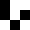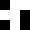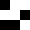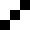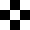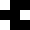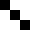我们可以说是$\,1$，因为被标记为$\,1\,$的图都是对称的；我们也可以说是$\,-1$，因为被标记为$\,-1\,$的图的左上角都是黑色的．这样就出现了不唯一的答案，也就是说，已知的数据集中没有足够的信息告诉我们那个是正确答案．

我们再来看一个更具体的二元分类例子，设$$f\colon\mathcal X=\{0,1\}^3\to\mathcal Y=\{\circ,\bullet\},$$并且我们已经知道了$\,f\,$在$\,\mathcal D\subsetneqq \mathcal X\,$上的取值$\,y_n=f(\bm x_n)$：$$\begin{array}{c|c}\bm x_n&y_n\\\hline(0,0,0)&\circ\\(0,0,1)&\bullet\\(0,1,0)&\bullet\\(0,1,1)&\circ\\(1,0,0)&\bullet\end{array}$$因为$\,f\,$是未知的，所以所有在$\,\mathcal D\,$中与$\,f\,$取值相同的函数都有可能是$\,f$，这样就会有$\,8\,$个可能的$\,f$：$$\begin{array}{c|c|c|c|c|c|c|c|c|c|c}\bm x_n&y_n&g&f_1&f_2&f_3&f_4&f_5&f_6&f_7&f_8\\\hline(0,0,0)&\circ&\circ&\circ&\circ&\circ&\circ&\circ&\circ&\circ&\circ\\(0,0,1)&\bullet&\bullet&\bullet&\bullet&\bullet&\bullet&\bullet&\bullet&\bullet&\bullet\\(0,1,0)&\bullet&\bullet&\bullet&\bullet&\bullet&\bullet&\bullet&\bullet&\bullet&\bullet\\(0,1,1)&\circ&\circ&\circ&\circ&\circ&\circ&\circ&\circ&\circ&\circ\\(1,0,0)&\bullet&\bullet&\bullet&\bullet&\bullet&\bullet&\bullet&\bullet&\bullet&\bullet\\\hline(1,0,1)&&?&\circ&\circ&\circ&\circ&\bullet&\bullet&\bullet&\bullet\\(1,1,0)&&?&\circ&\circ&\bullet&\bullet&\circ&\circ&\bullet&\bullet\\(1,1,1)&&?&\circ&\bullet&\circ&\bullet&\circ&\bullet&\circ&\bullet\end{array}$$这让我们开始怀疑机器学习的可行性．

如果我们坚持$\,f\,$是完全未知的，我们就无法从数据集中学到任何东西，所有我们应该对$\,f\,$作出一些假设．在解决这个问题之前，我们先来看另一件事情．

平面上有一堆两种颜色的点，我们想要知道蓝点在其中占的比例$\,\mu$．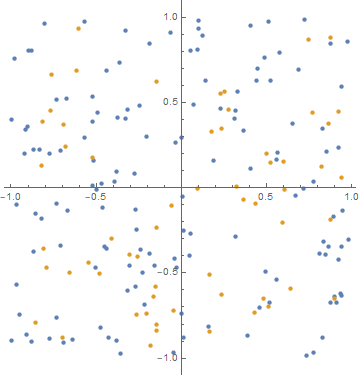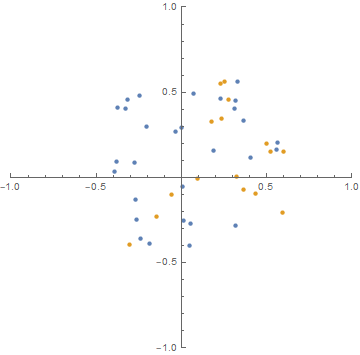现在我们尝试把抓点这件事与机器学习进行联系．对于每一个给定的假设函数$\,h\in\mathcal H$，将它与目标函数$\,f\,$不相等的可能性类比为蓝点的真实比例$\,\mu$，把$\,\mathcal X\,$类比为所有的蓝点和黄点，把$\,\mathcal D\,$类比为我们抓出来的那一把点，把使得$\,h(\bm x)\neq f(\bm x)\,$的$\,\bm x\,$类比为蓝点，使得$\,h(\bm x)=f(\bm x)\,$的$\,\bm x\,$类比为黄点．那么我们就可以把$\,h\,$在$\,\mathcal D\,$上的错误率$\,E_{\mathcal D}(h)\,$近似地作为在$\,\mathcal X\,$上的错误率$\,E_{\mathcal X}(h)$．这时仍然有霍夫丁不等式成立$$P[|E_{\mathcal X}(h)-E_{\mathcal D}(h)|>\varepsilon]\leqslant2\mathrm e^{-2\varepsilon^2N}.$$值得注意的是，对于一个固定的$\,h$，只保证$\,E_{\mathcal D}(h)\approx E_{\mathcal X}(h)\,$是不够的，因为我们无法保证它足够小．所以我们还应该使用一个新的数据集$\,\mathcal V\,$来检验$\,h\,$的错误率．

注意到在前面抓点的例子中，我们仍有可能抓出一把全是蓝色的点，即使这样的概率很小很小．此时我们当然不能说蓝点的比例为$\,100\%$．我们把这样一把点叫做坏样本．

当有多个假设函数时，设$$\mathcal H=\{h_1,h_2,\cdots,h_M\}.$$我们希望在$\,\mathcal H\,$中挑出一个最好的函数作为$\,g$，使得它与$\,f\,$很接近．如果$\,h_1\,$实际上是一个很好的函数，但是对它而言$\,\mathcal D\,$是一个坏样本，使得$\,E_{\mathcal D}(h_1)\,$很大，最终$\,h_1\,$没有被选为$\,g$；如果$\,h_2\,$实际上是一个很不好的函数，但是对它而言$\,\mathcal D\,$是一个坏样本，使得$\,E_{\mathcal D}(h_2)\,$很小，反而$\,h_2\,$被选为了$\,g$．可以看到，只要$\,\mathcal H\,$中有一个函数碰到坏样本，使得$$|E_{\mathcal X}(h_i)-E_{\mathcal D}(h_i)|>\varepsilon,$$就会对我们挑选最佳函数的过程造成影响．事实上，由于一个数据集只要对一个预测函数$\,h_i\,$是坏样本那么它就是坏样本，所以有
\begin{align*}P[\mathcal D \text{ is bad}]&=P\left[\bigvee_{i=1}^M\left(\mathcal D \text{ is bad for }h_i\right)\right]\\&\leqslant\sum_{i=1}^MP\left[\mathcal D \text{ is bad for }h_i\right]\\&\leqslant2M\mathrm e^{-2\varepsilon^2N}.\end{align*}因此对于有限个假设函数，只要数据量足够大，那么遇到坏样本的概率就会足够小．

## 至此，我们就说明了当数据集遵循概率上的某种分布时，机器学习是可行的．最后，我们再整理一下我们的逻辑顺序：首先我们指出机器学习不能找到一个与$\,f\,$一摸一样的函数$\,g$；然后用概率论的知识说明对于一个给定的$\,h$，$E_{\mathcal D}(h)\,$和$\,E_{\mathcal D}(h)\,$是可以很接近的；最后说明对于有限多个$\,h_i$，仍能保证$\,E_{\mathcal D}(h_i)\,$和$\,E_{\mathcal D}(h_i)\,$是足够接近．

参考资料 台湾大学《机器学习基石》